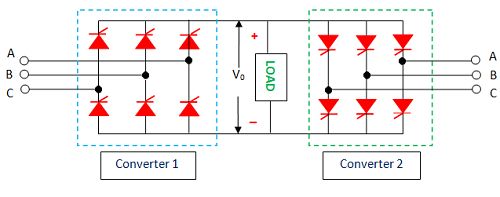## Search This Blog

### Three phase dual converter drive

• The schematic diagram of three phases dual converter d drive as shown in the figure.
• As per the circuit diagram converter, 1 allows motor control 1 and 4 quadrants whereas with converter 2 the operation in 2 and 3 quadrants obtained
• For reversing the polarity of motor generated emf for regeneration purpose, field circuit must be energized from a single phase or three phases full converter.Three phases dual converter dc drive When converter 1 or 2 is in operation Average output voltage, V0 =  Vt is =  3Vm l/ 2∏  * Cos  ɖ1  for 0<  ɖ1 <  ∏ With a 3 phase full converter in the filed circuit, Vf  =  3Vml/2∏ * Cos  ɖf  for 0<  ɖf <  ∏ In case of circulating types of dual converter, ɖ1  + ɖ2 =180 degree Application : Direction and speed control dc motors. Applicable wherever the reversible dc is required. Industrial variable speed dc drive.
• The schematic diagram of three phases dual converter d drive as shown in the figure.
• As per the circuit diagram converter, 1 allows motor control 1 and 4 quadrants whereas with converter 2 the operation in 2 and 3 quadrants obtained
• For reversing the polarity of motor generated emf for regeneration purpose, field circuit must be energized from a single phase or three phases full converter.Three phases dual converter dc drive When converter 1 or 2 is in operation Average output voltage, V0 =  Vt is =  3Vm l/ 2∏  * Cos  ɖ1  for 0<  ɖ1 <  ∏ With a 3 phase full converter in the filed circuit, Vf  =  3Vml/2∏ * Cos  ɖf  for 0<  ɖf <  ∏ In case of circulating types of dual converter, ɖ1  + ɖ2 =180 degree Application : Direction and speed control dc motors. Applicable wherever the reversible dc is required. Industrial variable speed dc drive.